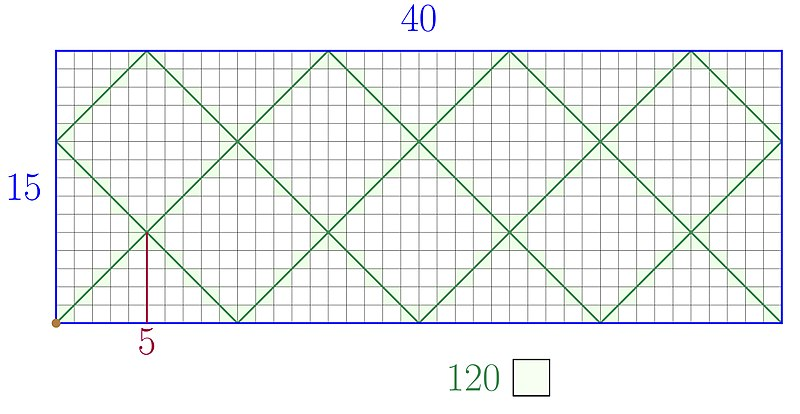# Arithmetic BilliardsTo find the least common multiple and the greatest common divisor of two natural numbers, construct a billiard table whose side lengths correspond to the two numbers (here, 15 and 40). Set a ball in one corner, fire it out at a 45-degree angle, and let it bounce around the table until it stops in a corner.

Now the least common multiple is the total number of unit squares traversed by the ball (here, 120).

And the greatest common divisor is the number of unit squares traversed by the ball before it reaches the first intersection (here, 5).

More details here.